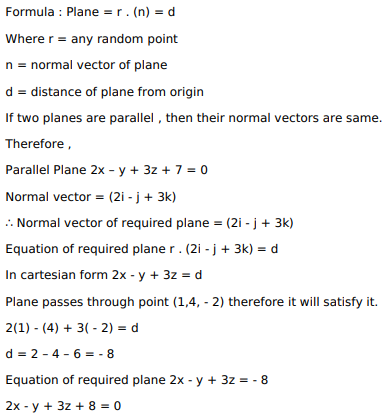# Find the equation of the plane passing through the pointQuestion:

Find the equation of the plane passing through the point $(1,4,-2)$ and parallel to the plane $2 x-y+3 z+7=0$.

Solution: